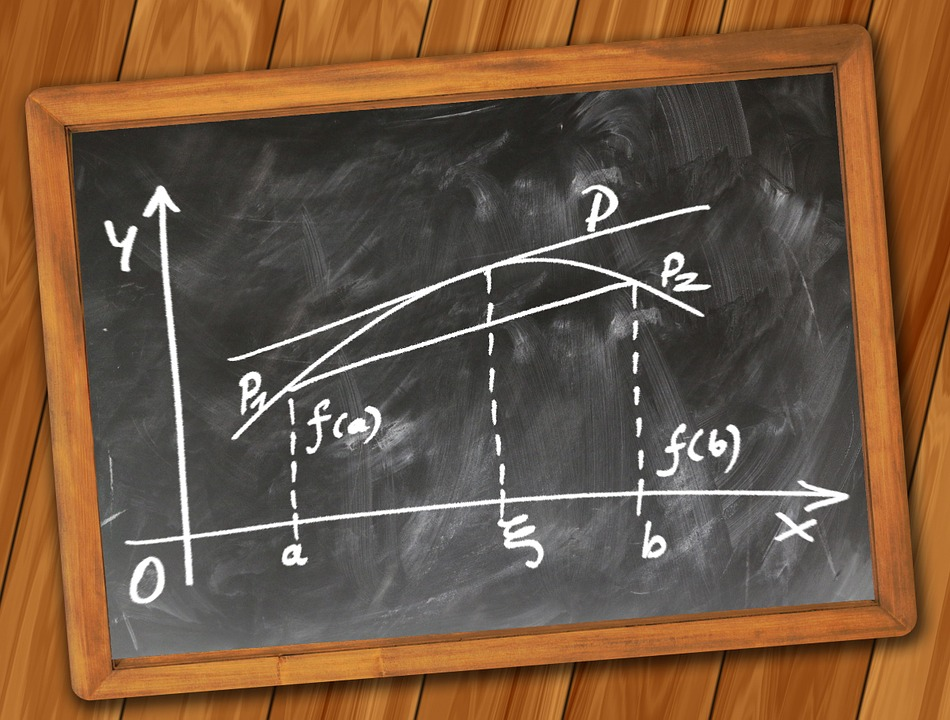## Distance Between Two Points in Cartesian Plane and Distance Formula Class 10th

Introduction In Coordinate Geometry, If there are two points situated in the Cartesian plane and we have to find the distance between them, then we can find the distance with the help of the Distance Formula. let’s see how we can find it. If Two Points are Situated on the Coordinate Axis (Whether on the …